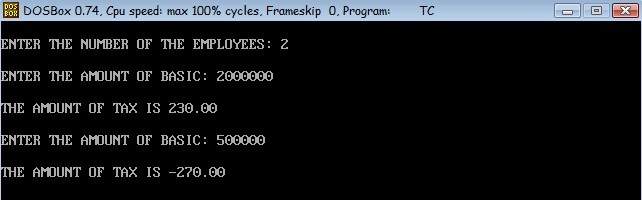# To calculate the tax using function in C

Levels of difficulty: / perform operation:

C program to calculate the tax of n given number of employees. Use a separate function to calculate the tax. Tax is 20% of basic if basic is less than 9000 otherwise tax is 25% of basic.

### C Program

```#include <stdio.h>
#include <conio.h>
void cal(void);
void main()
{
int i,n;
clrscr();
printf("\nENTER THE NUMBER OF THE EMPLOYEES: ");
scanf("%d",&n);
for(i=1;i<=n;i++)
cal();
getch();
}
void cal()
{
int basic;
float tax;
printf("\nENTER THE AMOUNT OF BASIC: ");
scanf("%d",&basic);
if(basic<9000)
tax=basic*20/100;
else
tax=basic*25/100;
printf("\nTHE AMOUNT OF TAX IS %0.2f\n",tax);
}
```

### Output